Courses

# Chemical Kinetics MCQ - 1 (Advanced)

## 25 Questions MCQ Test Chemistry for JEE Advanced | Chemical Kinetics MCQ - 1 (Advanced)

Description
This mock test of Chemical Kinetics MCQ - 1 (Advanced) for JEE helps you for every JEE entrance exam. This contains 25 Multiple Choice Questions for JEE Chemical Kinetics MCQ - 1 (Advanced) (mcq) to study with solutions a complete question bank. The solved questions answers in this Chemical Kinetics MCQ - 1 (Advanced) quiz give you a good mix of easy questions and tough questions. JEE students definitely take this Chemical Kinetics MCQ - 1 (Advanced) exercise for a better result in the exam. You can find other Chemical Kinetics MCQ - 1 (Advanced) extra questions, long questions & short questions for JEE on EduRev as well by searching above.
*Multiple options can be correct
QUESTION: 1

### Ozone decomposes according to the equation 2O3(g) → 3O2(g) Mechanism of the reaction is Step I : O3(g)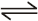O2(g) + O(g) (fast) Step II : O3(g) + O(g) → 2O2(g) (slow) Which of the following is correct ?

Solution: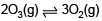Slowest step is rate determing step
rate  = K[O3][O]  .........(1)
Since, [O] is not in the original reaction hence it has to be eliminated.
From first step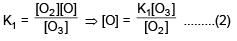Putting the value of [O] in the eq. (1)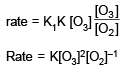*Multiple options can be correct
QUESTION: 2

### Consider the following case of competing 1st order reactions After the start of the reaction at t = 0, with only P, concentration of Q is equal to R at all times. The time in which all the three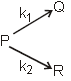concentration will be equal is given by

Solution: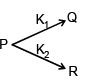Since [Q] = [R] all the times,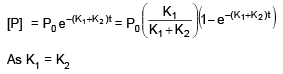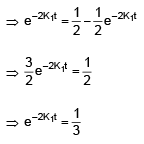Taking ln of both side
–2K1t = – ln3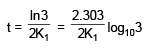As K1 = K2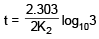*Multiple options can be correct
QUESTION: 3

### A substance undergoes first order decomposition. The decomposition follows two parallel first order reactions as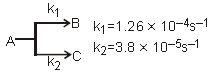The % distribution of B and C is ................ and .................. respectively.

Solution: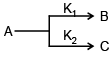K1 = 1.26 × 10–4 sec–1
K2 = 3.8 × 10–5 sec–1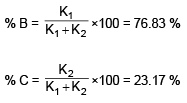*Multiple options can be correct
QUESTION: 4

Which of the following concepts are correct

Solution:

Energy of activation can never be negative Option (D) is incorrect.

*Multiple options can be correct
QUESTION: 5

The basic theory behind Arrhenious equation is that

Solution:

Only ans will be given

*Multiple options can be correct
QUESTION: 6

Which of the following statements are correct about half life period ?

Solution:

(A) For zero order reaction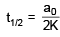⇒ t1/2 α a0 (initial concentration)
(B) For first order reaction
Average life = 1.44 × half life
(C) For IInd order reaction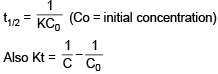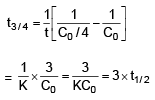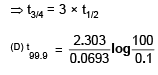t99.9  = 100 min

*Multiple options can be correct
QUESTION: 7

For a reaction : 2A + 2B → products, the rate law expression is r = k[A]2 [B]. Which of the following is/are correct?

Solution:

Rate = K[A]2[B]
⇒  rate w.r.t B = 1
Overall order of the reaction = 2 + 1 = 3
for a complex reaction the rate determing step is determined experimentally.

*Multiple options can be correct
QUESTION: 8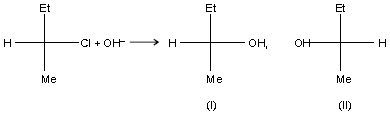Which of the following statements are correct ?

Solution:

In SN1 reaction, formation of carbocation takes place which is planar.
⇒ equimolar mixture of (I) and (II) will be formed in case of SN1.
In SN2 reaction Nucleophile will attack from the opposite to leaving group
⇒ Product (II) will be formed in case of SN2 reaction.

*Multiple options can be correct
QUESTION: 9

Rate constant k varies with temperature by equation log10k(min-1) = 5 – 2000/T. We can conclude

Solution: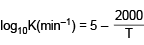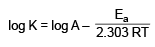log A = 5 ⇒ A = 105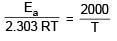Ea = 2000 × 2.303 × 8.314 cal
Ea = 9.212 Kcal

*Multiple options can be correct
QUESTION: 10

A reaction is catalysed by H+ ion. In presence of HA, rate constant is 2 × 10-3 min-1and in presence of HB rate constant is 1 × 10-3 min-1. HA and HB being strong acids, we may conclude that

Solution: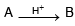Rate = K[H+][A]
Rate = K’[A]
If K’ has greater value for HA
⇒ [H+] has to be large
⇒ for that acid has be stronger
⇒ [HA] is strongers that HB
Also Relative strength = 2

QUESTION: 11

The elements which are good catalysts and have the ability to change their oxidation number are

Solution:
*Multiple options can be correct
QUESTION: 12

Statement-1 : The time of completion of reactions of type A → product (order <1) may be determined.

Statement-2 : Reactions with order ³ 1 are either too slow or too fast and hence the time of completion can not be determined.

Solution:

eg. Zero order reaction completion time can be determind for order > 1 time can be determined

*Multiple options can be correct
QUESTION: 13

Statement-1 : Temperature coefficient of an one step reaction may be negative.

Statement-2 : The rate of reaction having negative order with respect to a reactant decreases with the increase in concentration of the reactant.

Solution:

Ea > 0
If order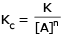*Multiple options can be correct
QUESTION: 14

Statement-1 : The overall rate of a reversible reaction may decrease with the increase in temperature.

Statement-2 : When the activation energy of forward reaction is less than that of backward reaction, then the increase in the rate of backward reaction is more than that of forward reaction on increasing the temperature.

Solution: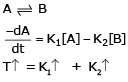*Multiple options can be correct
QUESTION: 15

Statement-1 : In a reversible endothermic reaction, Eact of forward reaction is higher than that of backward reaction

Statement-2 : The threshold energy of forward reaction is more than that of backward reaction

Solution: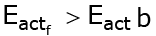∴ ΔH > 0

*Multiple options can be correct
QUESTION: 16

A hypothetical elementary reaction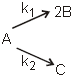where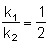Initially only 2 moles of A are present.

The total number of moles of A, B & C at the end of 50% reaction are

Solution: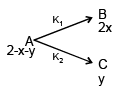2 – x – y = 1
⇒ x + y = 1  ............(1)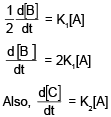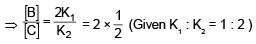⇒ [B]  = [C]
⇒ 2x = y  .............(2)
Solving (1) and (2)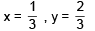Total moles after 50 % of the reaction
= 2 – x – y + 2x + y
= 2 + x = 7/3

*Multiple options can be correct
QUESTION: 17

A hypothetical elementary reactionwhere​ Initially only 2 moles of A are present.

Number of moles of B are

Solution:

No. of Moles of B = 2x
= 2/3
= 0.666

QUESTION: 18

The rate of a reaction increases significantly with increase in temperature. Generally, rates of reaction are doubled for every 10º rise in temperature. Temperature coefficient gives us an idea about the change in rate of a reaction for every 10º change in temperature.

Temperature coefficient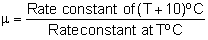Arrhenious gave an equation which describes rate constant k as a function of temperature is k = A –Ea/RT

where k is a rate constant

A is frequency factor or pre exponential factor

Ea is activation energy

T is temperature in kelvin and

R is universal gas constant

Equation when expressed in logarithmic form becomes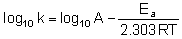Q.

For a reaction Ea = 0 and k = 3.2 × 105 s-1 at 325 K. The value of k at 335 K would be

Solution: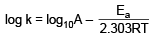When Ea = 0, k = 3.2 ×105 & it does not depend on T

QUESTION: 19

The rate of a reaction increases significantly with increase in temperature. Generally, rates of reaction are doubled for every 10º rise in temperature. Temperature coefficient gives us an idea about the change in rate of a reaction for every 10º change in temperature.

Temperature coefficientArrhenious gave an equation which describes rate constant k as a function of temperature is k = A –Ea/RT

where k is a rate constant

A is frequency factor or pre exponential factor

Ea is activation energy

T is temperature in kelvin and

R is universal gas constant

Equation when expressed in logarithmic form becomesQ.

For which of the following reactions k310/k300 would be maximum ?

Solution:

When Ea = is max,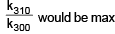QUESTION: 20

The rate of a reaction increases significantly with increase in temperature. Generally, rates of reaction are doubled for every 10º rise in temperature. Temperature coefficient gives us an idea about the change in rate of a reaction for every 10º change in temperature.

Temperature coefficientArrhenious gave an equation which describes rate constant k as a function of temperature is k = A –Ea/RT

where k is a rate constant

A is frequency factor or pre exponential factor

Ea is activation energy

T is temperature in kelvin and

R is universal gas constant

Equation when expressed in logarithmic form becomesQ.

Activation energies of two reaction are Ea and Ea with Ea > Ea. If the temperature of the reacting systems is increased from T1 to T2 (k` are rate constants at higher temperature).

Solution: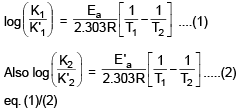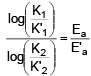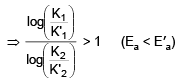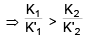QUESTION: 21

The rate of a reaction increases significantly with increase in temperature. Generally, rates of reaction are doubled for every 10º rise in temperature. Temperature coefficient gives us an idea about the change in rate of a reaction for every 10º change in temperature.

Temperature coefficientArrhenious gave an equation which describes rate constant k as a function of temperature is k = A –Ea/RT

where k is a rate constant

A is frequency factor or pre exponential factor

Ea is activation energy

T is temperature in kelvin and

R is universal gas constant

Equation when expressed in logarithmic form becomesQ.

For the reactions, following data is given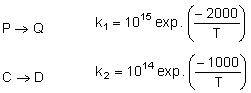Temperature at which k1 = k2 is

Solution: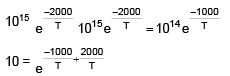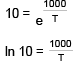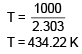QUESTION: 22

Match the column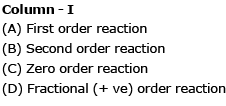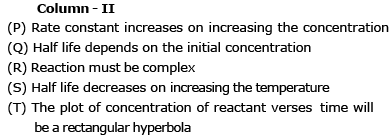Solution:
*Answer can only contain numeric values
QUESTION: 23

The activation energy of a reaction at a given temperature is found to be 2.303 RT J mol–1. The ratio of rate constant to the Arrhenius factor is

Solution: K=Ae^(-Ea/RT), K/A=10^(-Ea/2.303RT), now put the value of activation energy, k/A=10^-1 , so, k/A=0.1
*Answer can only contain numeric values
QUESTION: 24

An optically active compound A upon acid catalysed hydrolysis yield two optically active compound B and C by pseudo first order kinetics. The observed rotation of the mixture after 20 min was 5° while after completion of the reaction it was –20°. If optical rotation per mole of A, B & C are 60°, 40° & –80°. Calculate half life of the reaction.

Solution: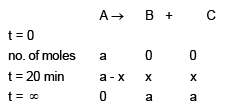Optical rotation reaction no. of moles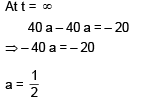At, t = 20 min
60 (a – x) + 40 x – 80 x = 5
60a – 100 x = 5
30 – 100 x = 5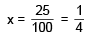No. of moles of A is becoming half after 20 min
∴ t1/2 = 20 min

*Answer can only contain numeric values
QUESTION: 25

Consider the following first order decomposition process: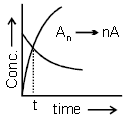Here, "t" corresponds to the time at which (1/6)th of reactant is decomposed. The value of "n" is

Solution: